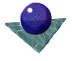Edges:
The Canny Edge DetectorThe Canny edge detector arises from the earlier work of Marr and Hildreth, who were concerned with modelling the early stages of human visual perception. In designing his edge detector he concentrated an ideal step edge, represented as a Sign function in one dimension, corrupted by an assumed Gaussian noise process. In practice this is not an exact model but it represents an approximation to the effects of sensor noise, sampling and quantisation. The approach was based strongly on convolution of the image function with Gaussian operators and their derivatives, so we shall consider these initially.

Considering the Gaussian function in one dimension, this may be expressed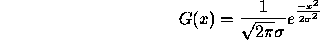and the first derivative is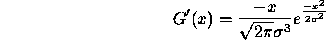and the second derivative is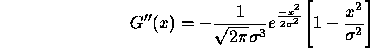Figure 3, below, shows the corresponding functions.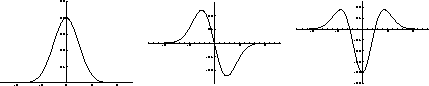Figure 3:   The Gaussian, first and second derivatives

The equivalent 2D functions are most easily expressed with respect to a polar coordinate system where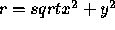represents the radial distance from the origin. The function is symmetrical and independent of. Thus,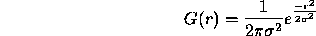and the first derivative is,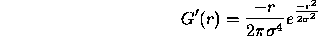and the second derivative is,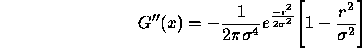Now consider an ideal step edge. When convolved with a Gaussian function the profile looks like the leftmost sketch of Figure 4, below. The next sketches show the first and second derivatives; the presence and location of the edge is marked by a peak and a zero crossing respectively.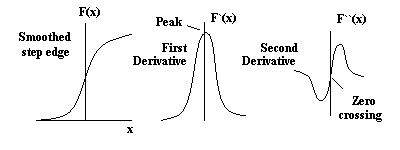Figure 4:   Successive edge derivatives

In fact, the first derivative of the image function convolved with a Gaussian,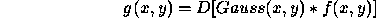is equivalent to the image function convolved with the first derivative of a Gaussian,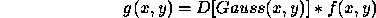Therefore, it is possible to combine the smoothing and detection stages into a single convolution in one dimension, either convolving with the first derivative of the Gaussian and looking for peaks, or with the second derivative and looking for zero crossings.

As an example, Figure 5, below, compares the response of a box filter and the first derivative of a Gaussian filter to a noisy step edge (from Canny's paper). The response of the Gaussian operator to the edge subjected to additive Gaussian noise is much improved with respect to the simple box operator ( which is similar to a Roberts or Sobel operator ). Edge detection could proceed by the simple thresholding of the data of the lowest figure, but in practice the final determination of the presence or absence of an edge is more complex, and it is common to include post-processing thinning and thresholding stages.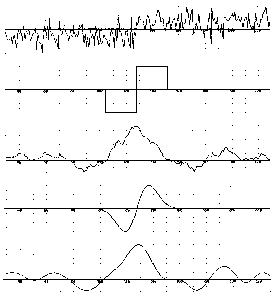Figure 5:   Comparing Gradient and DOG edge detection

The goals of the Canny Operator were stated explicitly.

(a)
Good Detection: the ability to locate and mark all real edges.
(b)
Good Localisation: minimal distance between the detected edge and real edge.
(c)
Clear Response: only one response per edge.

To fulfil these objectives, the edge detection process included the following stages.

Stage One - Image Smoothing

The image data is smoothed by a two dimensional Gaussian function of width specified by a user parameter. In practice, two dimensional convolution with large Gaussians takes a long time, so that in practice it is common to approximate this by two one dimensional Gaussians, one aligned with the x-axis, the other with the y axis. This produces two (rather than one) values at each pixel.

Stage Two - Differentiation

Assuming two dimensional convolution at stage 1, the smoothed image data is differentiated with respect to the x and y directions. It is possible to compute the gradient of the smooth surface of the convolved image function in any direction from the known gradient in any two directions.

Assuming the one dimensional approximation at stage one, then the values in the x-smoothed image array are convolved with a first derivative of a one dimensional Gaussian of identical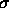aligned with y. Similarly, values in the y-smoothed image array are convolved with a first derivative of a one dimensional Gaussian of identicalaligned with x.

From the computed x and y gradient values, the magnitude and angle of the slope can be calculated from the hypoteneuse and arctangent in a similar manner to the Sobel operator.

Stage Three - Non-maximum Suppression

Having found the rate of intensity change at each point in the image, edges must now be placed at the points of maxima, or rather non-maxima must be suppressed. A local maximum occurs at a peak in the gradient function, or alternatively where the derivative of the gradient function is set to zero. However, in this case we wish to surpress non-maxima perpendicular to the edge direction, rather than parallel to (along) the edge direction, since we expect continuity of edge strength along an extended contour. (This assumption creates a problem at corners !)

Rather than perform an explicit differentiation perpendicular to each edge, another approximation is often used. Each pixel in turn forms the centre of a nine pixel neighbourhood. By interpolation of the surrounding discrete grid values, the gradient magnitudes are calculated at the neighbourhood boundary in both directions perpendicular to the centre pixel, as shown in Figure 6, below. If the pixel under consideration is not greater than these two values (i.e. non-maximum), it is suppressed.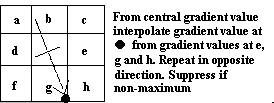Figure 6:   Non-maximum Suppression

Stage Four - Edge Thresholding

The thresholder used in the Canny operator uses a method called "hysteresis". Most thresholders used a single threshold limit, which means if the edge values fluctuate above and below this value the line will appear broken (commonly referred to as ``streaking''). Hysteresis counters streaking by setting an upper and lower edge value limit. Considering a line segment, if a value lies above the upper threshold limit it is immediately accepted. If the value lies below the low threshold it is immediately rejected. Points which lie between the two limits are accepted if they are connected to pixels which exhibit strong response. The likelihood of streaking is reduced drastically since the line segment points must fluctuate above the upper limit and below the lower limit for streaking to occur. Canny recommends the ratio of high to low limit be in the range two or three to one, based on predicted signal-to-noise ratios.

Figures 7 and 8 show examples of the Canny operator applied to a facial image at different scales and different hysteresis thresholds. Canny proposed a final processing stage, aggregation or feature synthesis. The general principle is to start from the smallest scale, then synthesise the larger scale outputs that would occur if they were the only edges present. Then compare the large scale output to the synthesis. Additional edges are marked if the large scale output is significantly greater than the synthetic prediction. The synthetic data was produced by convolving a Gaussian of large scale with the small scale edge data. The procedure is repeated to mark edges at the second scale which were not present at the first, then third to second and so on. Canny observed that `in the many cases the majority of edges were picked up by the smaller channel, and that later channels mark mostly shadow and shading edges, or edges between textured regions' (J. Canny, ``A computational approach to edge detection", IEEE Trans. on Pattern Analysis and Machine Intelligence, 8(6),pp679-698 (1986).Comments to: Sarah Price at ICBL.
(Last update: 4th July 1996)